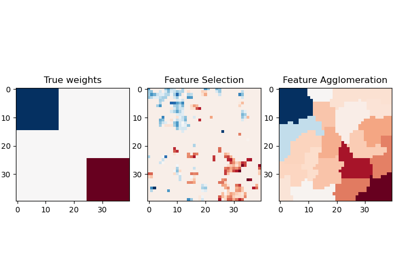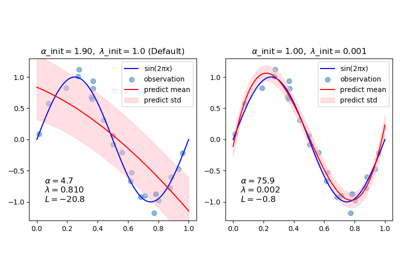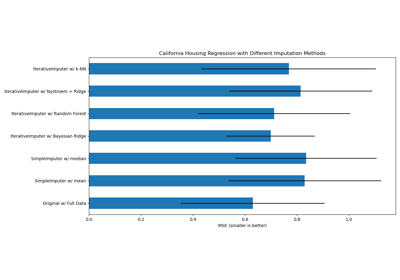# sklearn.linear_model.BayesianRidge¶

class sklearn.linear_model.BayesianRidge(*, n_iter=300, tol=0.001, alpha_1=1e-06, alpha_2=1e-06, lambda_1=1e-06, lambda_2=1e-06, alpha_init=None, lambda_init=None, compute_score=False, fit_intercept=True, normalize=False, copy_X=True, verbose=False)[source]

Bayesian ridge regression.

Fit a Bayesian ridge model. See the Notes section for details on this implementation and the optimization of the regularization parameters lambda (precision of the weights) and alpha (precision of the noise).

Read more in the User Guide.

Parameters
n_iterint, default=300

Maximum number of iterations. Should be greater than or equal to 1.

tolfloat, default=1e-3

Stop the algorithm if w has converged.

alpha_1float, default=1e-6

Hyper-parameter : shape parameter for the Gamma distribution prior over the alpha parameter.

alpha_2float, default=1e-6

Hyper-parameter : inverse scale parameter (rate parameter) for the Gamma distribution prior over the alpha parameter.

lambda_1float, default=1e-6

Hyper-parameter : shape parameter for the Gamma distribution prior over the lambda parameter.

lambda_2float, default=1e-6

Hyper-parameter : inverse scale parameter (rate parameter) for the Gamma distribution prior over the lambda parameter.

alpha_initfloat, default=None

Initial value for alpha (precision of the noise). If not set, alpha_init is 1/Var(y).

New in version 0.22.

lambda_initfloat, default=None

Initial value for lambda (precision of the weights). If not set, lambda_init is 1.

New in version 0.22.

compute_scorebool, default=False

If True, compute the log marginal likelihood at each iteration of the optimization.

fit_interceptbool, default=True

Whether to calculate the intercept for this model. The intercept is not treated as a probabilistic parameter and thus has no associated variance. If set to False, no intercept will be used in calculations (i.e. data is expected to be centered).

normalizebool, default=False

This parameter is ignored when fit_intercept is set to False. If True, the regressors X will be normalized before regression by subtracting the mean and dividing by the l2-norm. If you wish to standardize, please use StandardScaler before calling fit on an estimator with normalize=False.

copy_Xbool, default=True

If True, X will be copied; else, it may be overwritten.

verbosebool, default=False

Verbose mode when fitting the model.

Attributes
coef_array-like of shape (n_features,)

Coefficients of the regression model (mean of distribution)

intercept_float

Independent term in decision function. Set to 0.0 if fit_intercept = False.

alpha_float

Estimated precision of the noise.

lambda_float

Estimated precision of the weights.

sigma_array-like of shape (n_features, n_features)

Estimated variance-covariance matrix of the weights

scores_array-like of shape (n_iter_+1,)

If computed_score is True, value of the log marginal likelihood (to be maximized) at each iteration of the optimization. The array starts with the value of the log marginal likelihood obtained for the initial values of alpha and lambda and ends with the value obtained for the estimated alpha and lambda.

n_iter_int

The actual number of iterations to reach the stopping criterion.

X_offset_float

If normalize=True, offset subtracted for centering data to a zero mean.

X_scale_float

If normalize=True, parameter used to scale data to a unit standard deviation.

Notes

There exist several strategies to perform Bayesian ridge regression. This implementation is based on the algorithm described in Appendix A of (Tipping, 2001) where updates of the regularization parameters are done as suggested in (MacKay, 1992). Note that according to A New View of Automatic Relevance Determination (Wipf and Nagarajan, 2008) these update rules do not guarantee that the marginal likelihood is increasing between two consecutive iterations of the optimization.

References

D. J. C. MacKay, Bayesian Interpolation, Computation and Neural Systems, Vol. 4, No. 3, 1992.

M. E. Tipping, Sparse Bayesian Learning and the Relevance Vector Machine, Journal of Machine Learning Research, Vol. 1, 2001.

Examples

>>> from sklearn import linear_model
>>> clf = linear_model.BayesianRidge()
>>> clf.fit([[0,0], [1, 1], [2, 2]], [0, 1, 2])
BayesianRidge()
>>> clf.predict([[1, 1]])
array([1.])


Methods

 fit(X, y[, sample_weight]) Fit the model get_params([deep]) Get parameters for this estimator. predict(X[, return_std]) Predict using the linear model. score(X, y[, sample_weight]) Return the coefficient of determination $$R^2$$ of the prediction. set_params(**params) Set the parameters of this estimator.
fit(X, y, sample_weight=None)[source]

Fit the model

Parameters
Xndarray of shape (n_samples, n_features)

Training data

yndarray of shape (n_samples,)

Target values. Will be cast to X’s dtype if necessary

sample_weightndarray of shape (n_samples,), default=None

Individual weights for each sample

New in version 0.20: parameter sample_weight support to BayesianRidge.

Returns
selfreturns an instance of self.
get_params(deep=True)[source]

Get parameters for this estimator.

Parameters
deepbool, default=True

If True, will return the parameters for this estimator and contained subobjects that are estimators.

Returns
paramsdict

Parameter names mapped to their values.

predict(X, return_std=False)[source]

Predict using the linear model.

In addition to the mean of the predictive distribution, also its standard deviation can be returned.

Parameters
X{array-like, sparse matrix} of shape (n_samples, n_features)

Samples.

return_stdbool, default=False

Whether to return the standard deviation of posterior prediction.

Returns
y_meanarray-like of shape (n_samples,)

Mean of predictive distribution of query points.

y_stdarray-like of shape (n_samples,)

Standard deviation of predictive distribution of query points.

score(X, y, sample_weight=None)[source]

Return the coefficient of determination $$R^2$$ of the prediction.

The coefficient $$R^2$$ is defined as $$(1 - \frac{u}{v})$$, where $$u$$ is the residual sum of squares ((y_true - y_pred) ** 2).sum() and $$v$$ is the total sum of squares ((y_true - y_true.mean()) ** 2).sum(). The best possible score is 1.0 and it can be negative (because the model can be arbitrarily worse). A constant model that always predicts the expected value of y, disregarding the input features, would get a $$R^2$$ score of 0.0.

Parameters
Xarray-like of shape (n_samples, n_features)

Test samples. For some estimators this may be a precomputed kernel matrix or a list of generic objects instead with shape (n_samples, n_samples_fitted), where n_samples_fitted is the number of samples used in the fitting for the estimator.

yarray-like of shape (n_samples,) or (n_samples, n_outputs)

True values for X.

sample_weightarray-like of shape (n_samples,), default=None

Sample weights.

Returns
scorefloat

$$R^2$$ of self.predict(X) wrt. y.

Notes

The $$R^2$$ score used when calling score on a regressor uses multioutput='uniform_average' from version 0.23 to keep consistent with default value of r2_score. This influences the score method of all the multioutput regressors (except for MultiOutputRegressor).

set_params(**params)[source]

Set the parameters of this estimator.

The method works on simple estimators as well as on nested objects (such as Pipeline). The latter have parameters of the form <component>__<parameter> so that it’s possible to update each component of a nested object.

Parameters
**paramsdict

Estimator parameters.

Returns
selfestimator instance

Estimator instance.

## Examples using sklearn.linear_model.BayesianRidge¶Feature agglomeration vs. univariate selection¶Curve Fitting with Bayesian Ridge Regression¶Bayesian Ridge Regression¶Imputing missing values with variants of IterativeImputer¶